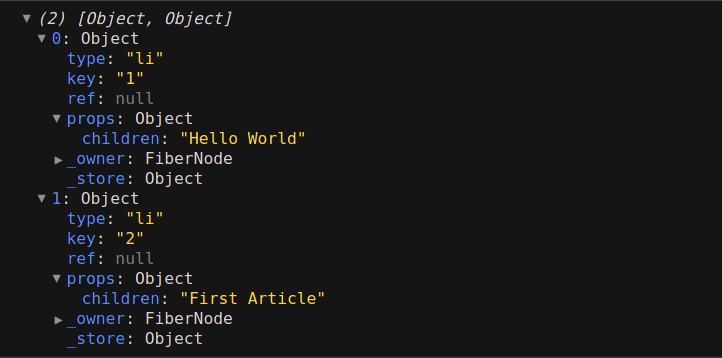# Amit Merchant

A blog on PHP, JavaScript, and more

# Array.map() vs Array.forEach() in JavaScript

Amit Merchant · February 25, 2022 ·

When it comes to traversing arrays in JavaScript, there are essentially two ways using which you can do this.

• To use `Array.map()`
• To use `Array.forEach()`

Both methods let you traverse an array and do things on the array elements. But there are some key differences between these methods that you need to keep in mind.

So, depending upon the situation, you would need to decide which method you should use among these two.

In this article, I’ll explain both of these methods and use-cases in which you can use them.

## The `Array.map()` method

The `map()` method lets you create a new array populated with the results of calling a provided callback function on every element in the calling array.

Here’s what the definition of the `map()` method looks like.

``````map(function(element, index, array) {
/* ... */
}, thisArg)
``````

As you can tell, the callback function inside `map()` will always be invoked with three arguments.

• The value of the element
• The index of the element
• The Array object being traversed

If we want to explicitly provide the `this` value to the method, we can do it by using the `thisArg` argument.

So, let’s say there’s an array called `numbers`, we can traverse through the array using the `map()` method like so.

``````const numbers = [1, 2, 3, 4];

// receive each element of array
// then multiply it times two
// map then returns a new array
const mapNumbers = numbers.map(function(number) {
return number * 2;
});

console.log(mapNumbers);
// [2, 4, 6, 8]
``````

As you can tell, the `map()` method would traverse through the `numbers` array, invoke the callback function provided on each array element, and return a brand new array.

One thing to note here is, the `map()` does not mutate the original array. So, the numbers array remains the same.

### When to use `Array.map()`

So, the question is when to use `Array.map()`?

The answer is, you should use the `map()` method in situations where you would be using the returned array by the method.

Take the following example of a React component.

``````export default function App() {
const posts = [
{
id: 1,
title: "Hello World",
content: "Welcome to amitmerchant.com!"
},
{
id: 2,
title: "First Article",
content: "My first article."
}
];

const sidebar = posts.map((post) => (
<li key={post.id}>{post.title}</li>
));

console.log(sidebar);

return (
<div>
<ul>
{sidebar}
</u>
</div>
);
}
``````

As you can tell, in this example, we have an array of objects called `posts` that we want to render. So, in this case, we can use the `map()` method since we want the returned array to be assigned to the `sidebar` variable.

If we log the `sidebar` variable to the console, we can see an array of objects that looks like so.React then loops over this array and use `props.children` to render the desired output.

So, the `map()` should be your “go-to” method in situations like this.

### When not to use `Array.map()`

When not to use `Array.map()`. The answer is simple. You should not reach for this method if you aren’t using the returned array by it. You should avoid using `map()` altogether.

## The `Array.forEach()` method

The `forEach()` method lets you traverse through the array and executes a provided function once for each array element exactly like the `map()` method. But there are a few differences.

• It doesn’t return a new array.
• It will always return the value `undefined`.

Here’s what the definition of the `forEach()` method looks like.

``````forEach(function(element, index, array) {
/* ... */
}, thisArg)
``````

As you can tell, the callback function inside `forEach()` will always be invoked with three arguments.

• The value of the element
• The index of the element
• The Array object being traversed

If we want to explicitly provide the `this` value to the method, we can do it by using the `thisArg` argument.

Here’s a simple example that demonstrates the use of the `forEach()` method.

``````const numbers = [1, 2, 3, 4];
const mapNumbers = [];

// receive each element of array
// then multiply it times two
// and then logs it in the console
numbers.forEach(function(number) {
mapNumbers.push(number * 2);
});

console.log(mapNumbers);
// [ 2, 4, 6, 8 ]
``````

As you can tell, since the `forEach()` method doesn’t return a new array, we need to manually push the array elements to the newly created array inside the callback function.

Also note that, we are effectively mutating the `mapNumbers` array here unlike the `map()` method which not always a good practice.

### When to use `Array.forEach()`

You can always reach for the `forEach()` method when you only want to do something with the array elements. For instance, in the case when you only want to print the elements of an array.

One more use case is to use it at the end of the chain when you want to perform some side-effects (such as logging).

### When not to use `Array.forEach()`

You should easily avoid using the `forEach()` method when you need to have the newly constructed array back since the method would always return the `undefined` value.

So, you can not use `forEach` when you want to render array elements inside a React component.

👋 Hi there! I'm Amit. I write articles about all things web development. If you like what I write and want me to continue doing the same, I would like you buy me some coffees. I'd highly appreciate that. Cheers!My Tiny YouTube Channel ⟶